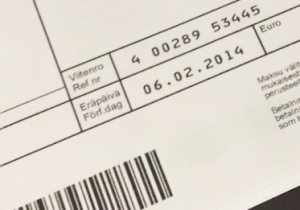Several years ago I had to implement a payment system where end users would receive invoices including reference numbers.

However, those numbers are commonly used nowadays I have never needed to implement the same functionality again since then - until recently when a few weeks ago a customer required it for an e-commerce solution.

Back then I have been working for another employee and therefore didn’t have any code base now I could refer to.

### Algorithm for calculating the check digit

Now the algorithm to create the reference number isn’t difficult after all when you know where to find the rules. “Viitenumero” is the Finnish word for “reference number” and one place to find the algorithm is here. [The algorithm might sound cryptic at first but don’t worry it’s just Finnish and besides that the algorithm itself is very easy to understand.]

Basically, you will have to calculate the check digit for a chosen basic number (3-19 digits):

• the digits in the basic number are multiplied by the weights 7, 3, 1, 7, 3, 1,… from right to left
• the products of each multiplications are added up
• the resulting sum is deducted from the next ten
• this calculated check digit will be added to the basic number
• in case the resulting difference is 10 the check digit will be set to 0

### Example showing how the check digit is calculated

A basic number 12345 would result in a reference number of 123453 with the following steps.

 Basic number 1 2 3 4 5 Weights from right to left 3 7 1 3 7 The products are added together 3 14 3 12 35 67 The following 10 70 From which the added sum is subtracted 70-67 Difference/Check digit 3

The resulting check digit 3 will be added to the basic number. There is one special case when the difference is 10. Then the check digit needs to be set to 0.

To just get a picture how those numbers are created and if you don’t need to create them automatically you could generate a batch of reference numbers from a bank site (other banks have similar calculators).

However, manually creation might be a solution for some situations in this case I needed to generate the numbers automatically every time a user places an order. I skip the part where I create unique base numbers here now. But you will need to think which option fits best and if you need one unique reference number per customer (i.e. used by some credit card bills) or increasing reference numbers for every new invoice (i.e. usually used by online orders).

But now enough about the theoretical part and let’s see how this could be implemented.

``````public int CalculateCheckSum(string referenceNumberBase)
{
int checkDigit = -1;

int[] multipliers = new int[] { 7, 3, 1 };
int multiplierIndex = 0;
int sum = 0;

for (int i = referenceNumberBase.Length - 1; i >= 0; i--)
{
if (multiplierIndex == 3)
{
multiplierIndex = 0;
}

sum += Convert.ToInt32(referenceNumberBase[i].ToString()) * multipliers[multiplierIndex];
multiplierIndex++;
}

checkDigit = 10 - sum % 10;

if (checkDigit == 10)
{
checkDigit = 0;
}

return checkDigit;
}
``````

The resulting reference number should be displayed in 5-digits blocks starting from the right for better readability. A basic implementation you can see below:

``````private string ImproveReadability(string text, int numberOfCharactersPerBlock)
{
return Regex.Replace(text, ".{" + numberOfCharactersPerBlock.ToString() + "}", " \$0", RegexOptions.RightToLeft);
}
``````

When calling the above method with 123456789012 you would get 12 34567 89012.

``````ImproveReadability("123456789012", 5);
``````

## Testing generated reference numbers

And to insure our method works as expected let’s add a few tests to validate our implementation of the algorithm works as expected. Following the TDD practice you might want to actually create the tests before the real implementation but at the time of writing this blog post all test and production code has already been implemented and therefore I have appended the tests at the end.

``````[TestFixture]
public class ReferenceNumberTests
{
[Test]
public void Ensure_reference_numbers_are_valid()
{
ReferenceNumber referenceNumber = new ReferenceNumber();

//input must be at least 3 characters and can contain maxium 19 characters

Assert.AreEqual(9, referenceNumber.CalculateCheckSum("100"));
Assert.AreEqual(2, referenceNumber.CalculateCheckSum("101"));
Assert.AreEqual(5, referenceNumber.CalculateCheckSum("102"));
Assert.AreEqual(6, referenceNumber.CalculateCheckSum("1001"));
Assert.AreEqual(0, referenceNumber.CalculateCheckSum("12345678"));

// algorithm difference is 10 --> therefore final checksum must be 0
Assert.AreEqual(0, referenceNumber.CalculateCheckSum("011"));
}

[Test]
public void Ensure_reference_numbers_are_in_range()
{
ReferenceNumber referenceNumber = new ReferenceNumber();

// lower end
Assert.AreEqual(true, referenceNumber.IsInRange("001"));

// upper end
Assert.AreEqual(true, referenceNumber.IsInRange("9999999999999999999"));
}

[Test]
public void Expect_reference_number_out_of_range()
{
ReferenceNumber referenceNumber = new ReferenceNumber();

// below lower end
Assert.AreEqual(false, referenceNumber.IsInRange("99"));

// above upper end
Assert.AreEqual(false, referenceNumber.IsInRange("10000000000000000000"));
}
}
``````